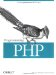3.2 Defining a Function

To define a function, use the following syntax:

function [&] function_name ( [ parameter [, ... ] ] ) {   statement list }

The statement list can include HTML. You can declare a PHP function that doesn't contain any PHP code. For instance, the column( ) function simply gives a convenient short name to HTML code that may be needed many times throughout the page:

<? function column(  ) { ?> </td><td> <? } ?>

The function name can be any string that starts with a letter or underscore followed by zero or more letters, underscores, and digits. Function names are case-insensitive; that is, you can call the sin( ) function as sin(1), SIN(1), SiN(1), and so on, because all these names refer to the same function.

Typically, functions return some value. To return a value from a function, use the return statement: put return expr inside your function. When a return statement is encountered during execution, control reverts to the calling statement, and the evaluated results of expr will be returned as the value of the function. Although it can make for messy code, you can actually include multiple return statements in a function if it makes sense (for example, if you have a switch statement to determine which of several values to return).

If you define your function with the optional ampersand before the name, the function returns a reference to the returned data rather than a copy of the data.

Let's take a look at a simple function. Example 3-1 takes two strings, concatenates them, and then returns the result (in this case, we've created a slightly slower equivalent to the concatenation operator, but bear with us for the sake of example).

Example 3-1. String concatenation
function strcat(\$left, \$right) {   \$combined_string = \$left . \$right;   return \$combined_string; }

The function takes two arguments, \$left and \$right. Using the concatenation operator, the function creates a combined string in the variable \$combined_string. Finally, in order to cause the function to have a value when it's evaluated with our arguments, we return the value \$combined_string.

Because the return statement can accept any expression, even complex ones, we can simplify the program as shown in Example 3-2.

Example 3-2. String concatenation redux
function strcat(\$left, \$right) {   return \$left . \$right; }

If we put this function on a PHP page, we can call it from anywhere within the page. Take a look at Example 3-3.

Example 3-3. Using our concatenation function
<?php  function strcat(\$left, \$right) {    return \$left . \$right;  }  \$first = "This is a ";  \$second = " complete sentence!";  echo strcat(\$first, \$second); ?>

When this page is displayed, the full sentence is shown.

This function takes in an integer, doubles it, and returns the result:

function doubler(\$value) {   return \$value << 1; }

Once the function is defined, you can use it anywhere on the page. For example:

<?= 'A pair of 13s is ' . doubler(13); ?>

You can nest function declarations, but with limited effect. Nested declarations do not limit the visibility of the inner-defined function, which may be called from anywhere in your program. The inner function does not automatically get the outer function's arguments. And, finally, the inner function cannot be called until the outer function has been called.

function outer (\$a) {   function inner (\$b) {     echo "there \$b";   }   echo "\$a, hello "; } outer("well"); inner("reader"); well, hello there readerProgramming PHP
ISBN: 1565926102
EAN: 2147483647
Year: 2007
Pages: 168

Similar book on Amazon